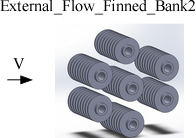Contents - Index

External_Flow_Finned_Bank2Procedure External_Flow_Finned_Bank2(Fluid\$, T, P, V, N_L, D_t, S_T, S_L, D_f, th_f, p_f: h, DELTAp, Nusselt, Re) calculates the average heat transfer coefficient, and total pressure drop over a staggered bank of finned tubes.  Properties are evaluated at the temperature and pressure provided and the units of the inputs and outputs correspond to the settings in EES.

Inputs

Fluid\$ can be any fluid in the EES data base.   The fluid can be an ideal gas, a real fluid, a brine, or an incompressible fluid in the Solid-Liquid_Props fluids library.

T - the fluid temperature in [C], [K], [F], or [R].

P -  pressure can be in [Pa], [kPa], [bar], [MPa], [atm], or [psia].   (Note that for brines, this parameter provides the concentration in % instead of pressure.)

V  - approach, free stream velocity of flow in [m/s] or [ft/min]

N_L - the number of rows of tubes

D_t -   tube diameter in [m] or [ft]

S_T is the transverse tube pitch in [m] or [ft]

S_L is the longitudinal tube pitch in [m] or [ft]

D_f is the outer diameter of the fin, in [m] or [ft]

th_f is the thickness of the fin in [m] or [ft]

p_f is the fin pitch (distance between fins) in [m] or [ft]

Outputs

h -  average heat transfer coefficient in [W/m^2-K] or [Btu/hr-ft^2-R]

deltap - total pressure drop in the pressure units set in the EES Unit System dialog

Nusselt - average Nusselt number [-]

Re - Reynolds number [-]

Notes

The procedure uses correlations and correction factors for pressure drop provided by Yudin and Tokhtarova (1974) as presented in the Heat Exchanger Design Handbook (2008).  The correlation for heat transfer is from PFR (2008).  The correlations for pressure drop apply from Re> 2.2e3.

Example

\$UnitSystem SI Mass J K Pa

Fluid\$='Air'

T = 400 [K]

P=101325 [Pa]

V=25 [m/s]

N_L=10

D_t=0.005 [m]

S_T=0.025 [m]

S_L=0.02 [m]

D_f=0.015 [m]

th_f=0.002 [m]

p_f=0.005 [m]

Call external_flow_finned_bank2(Fluid\$, T,  P, V, N_L, D_t, S_T, S_L, D_f, th_f, p_f: h, DELTAp, Nusselt, Re)

{Solution: h=296.2 [W/m^2-K],  DELTAp=2195 [Pa], Nusselt=45.1 [-], Re=6016 [-]}